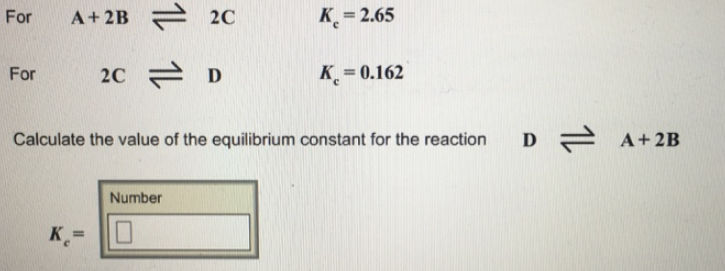# Problem: For A + 2B ⇌ 2C           K  c = 2.65 For 2C ⇌ D                                        K      c = 0.162 Calculate the value of the equilibrium constant for the reaction D ⇌ A + 2B

###### FREE Expert Solution
81% (158 ratings)###### Problem Details

For A + 2B ⇌ 2C           K  c = 2.65

For 2C ⇌ D                                        K      c = 0.162

Calculate the value of the equilibrium constant for the reaction D ⇌ A + 2B